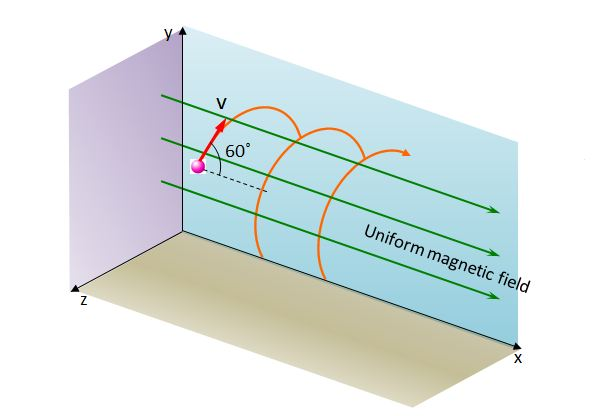# Helical motion of a particleThe above shows the path of a charged particle in an $xyz$-space where a uniform magnetic field exists in the $+x$ direction. When the particle is thrown at a speed of $v,$ forming a $60°$ angle with the direction of the magnetic field, its path takes a helical motion as shown above. It takes $T$ seconds for the particle to complete a turn around. Which of the following statements is correct?

a) The particle is a positive electric charge.

b) The direction of the magnetic force is perpendicular to the direction of the magnetic field.

c) The particle moves $vT$ in the $+x$ direction in $T$ seconds.

×

Problem Loading...

Note Loading...

Set Loading...